Browse by Topic
Related Topics

# Biggest Little Polyhedron—New Solutions in Combinatorial Geometry

In many areas of mathematics, 1 is the answer. Squaring a number above or below 1 results in a new number that is larger or smaller. Sometimes, determining whether something is “big” is based on whether a largest dimension is greater than 1. For instance, with sides of length 13,800 km, Saturn’s hexagon would be considered big. A “little polygon” is defined as a polygon where 1 is the maximum distance between vertices. In 1975, Ron Graham found the biggest little hexagon, which has more area than the regular hexagon, as shown below. The red diagonals have length 1. All other diagonals (not drawn) are smaller than 1.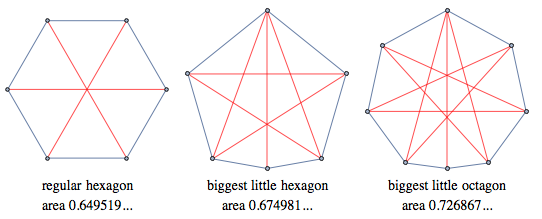I’ve often wondered what the biggest little polyhedra would look like. Mathematica 10 introduced Volume[ConvexHullMesh[points]], so I thought I could solve the problem by picking points at random. Below is some code for picking, calculating, and showing a random little polyhedron. If the code is run a thousand times, one of the solutions will be better than the others. Here, I ran it three times. One of these three solutions is (probably) better than the other two.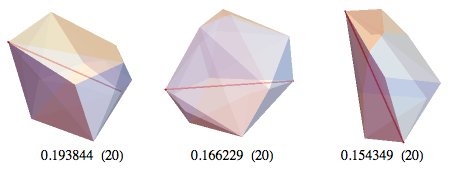With randomly selected points, images like the following emerge from the better solutions. I posted these on Wolfram Community under the discussion Biggest Little Polyhedra, and got some useful comments from Robin Houston and Todd Rowland. I thought of using results from “Visualizing the Thomson Problem” for solutions. In the Thomson problem, electrons repel each other on a sphere. Twelve repelling points move to the vertices of an icosahedron, which is inefficient for BLP, since all the longest distances pass through the center of the bounding sphere, just like the regular hexagon in the polygon case. I modified the Thomson code so that points repelled each other and all polar opposites, and that gave good starting values.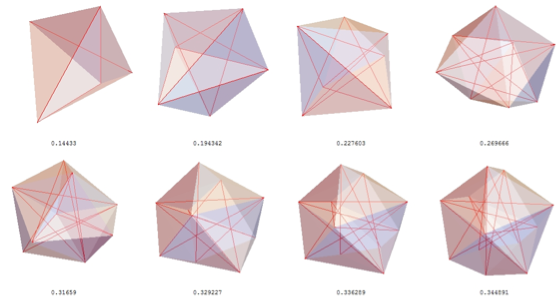Four points need a regular tetrahedron, with volume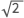/12= 0.117851.
Five points need a unit equilateral triangle with a perpendicular unit line, with volume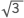/12 = 0.1443375; solved in 1976 .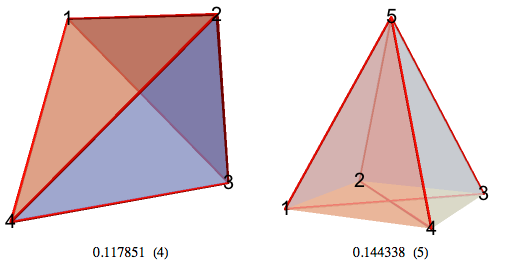I’ll use the name 6-BLP for the biggest little polyhedron on 6 points. In 2003, the volume for 6-BLP was solved to four decimals of accuracy [2, 3]. Graphics for 6-BLP and 7-BLP are below, with red lines for the unit diagonals.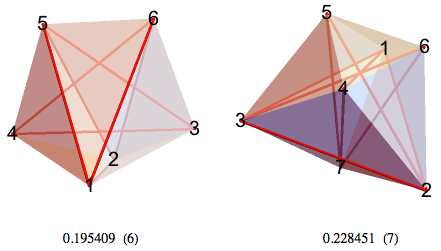To find these on my own, I first picked the best solutions out of a thousand tries, then used simulated annealing to improve the solutions. For each of the points in the good solution, I introduced a tiny bit of randomness to try to find a better solution, thousands of times. Then I introduced a tinier bit of randomness, over and over again. Some of these solutions seemed to go to a symmetrical solution. For example, with seven points, the best solution seemed to be gradually drifting toward this polyhedron, with a value of r of about a half, which represents the relative size of the upper triangle △456.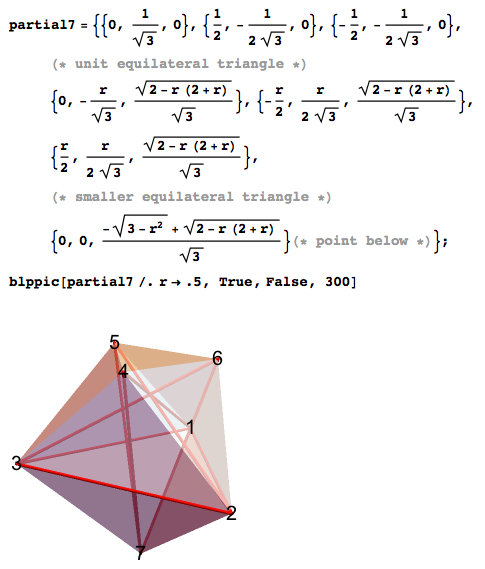The exact volume can be determined by the tetrahedra defined by the points {{2,3,4,7}, {2,4,6,7}, {5,4,7,6}}, with the volumes of the first two being tripled for symmetry. Look at the volumes of the tetrahedra, and switch any two numbers in a tetrahedron with negative volume.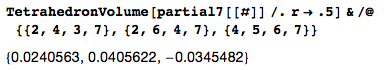After changing the parity of the last tetrahedron, we can calculate the exact r that gives the exact optimal volume. In the same way, we’ll also solve a few others.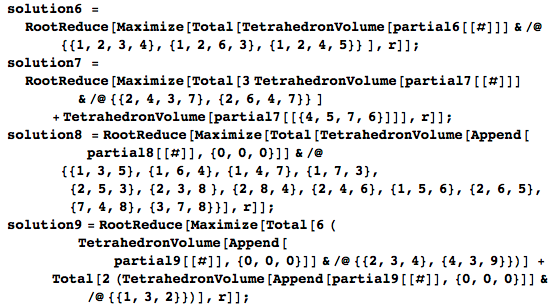The solution for 16-BLP takes more than a minute, so I’ve separated it out.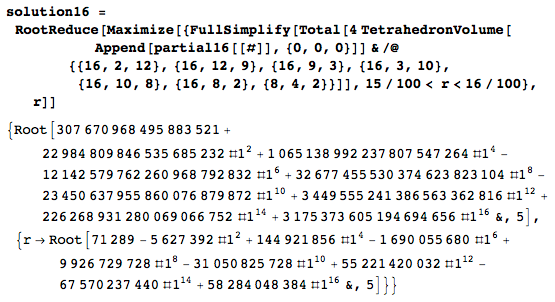The first value in the solutions is the optimal volume as a Root object, and the second is the optimal value of r. Here’s a cleaner table of values.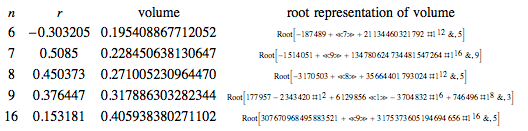That is far beyond anything I could have solved by hand. With random selection, annealing, symmetry spotting, Solve[], and Maximize[], I was also able to find the exact n-BLP (biggest little polygon) for n = 6, 7, 8, 9, and 16.

Here are a few views of the 8-BLP, with the red tubes showing unit-length diagonals.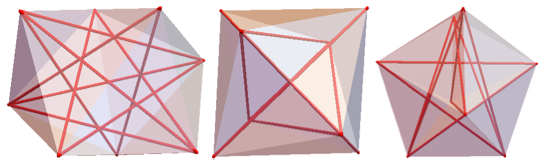Some views of 9-BLP: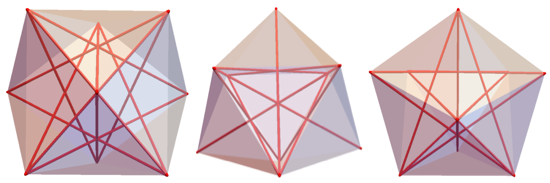Some views of 16-BLP: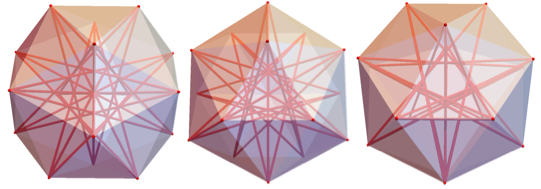The labeled 8-BLP below features perpendicular unit lines 1-2 and 3-4 above and below the origin. The labeled 9-BLP below features stacked triangles △123, △456, and △789.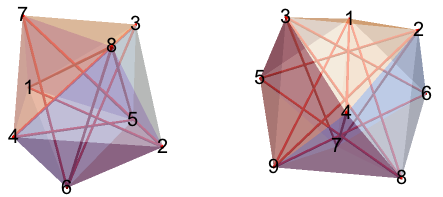The labeled 16-BLP below features a truncated tetrahedron on points 1-12 and added points 13-16.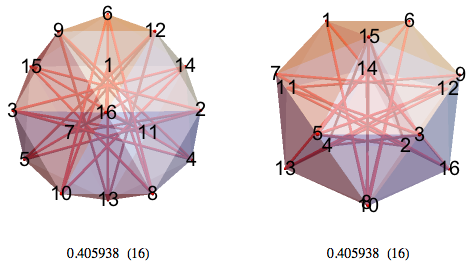Fairly complicated, right? With sphere point picking, random numbers –Pi to Pi and –1 to 1 can produce randomly distributed points on a unit sphere. Points on a unit sphere can be mapped back to points in the (–Pi to Pi, –1 to 1) rectangle. With the solutions for 8, 9, and 16, here’s what happens.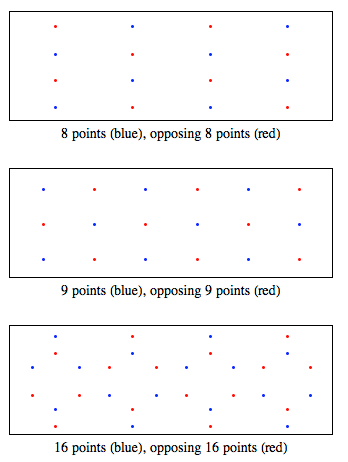For 10-BLP, I haven’t been able to find an exact solution, but I did find a numerical solution to any desired level of accuracy. If anyone can find a root object for this, let me know. Open up the notebook version to see a rather difficult equation in the Initialization section.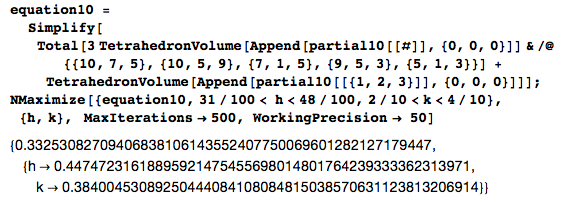Here’s a labeled view of 10-BLP from two different perspectives.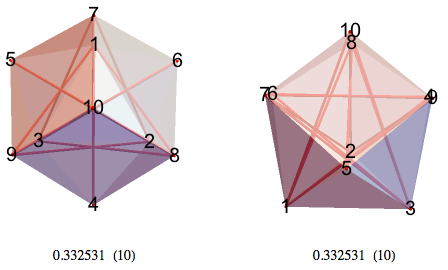In a similar fashion, a numerical solution for 11-BLP can be found.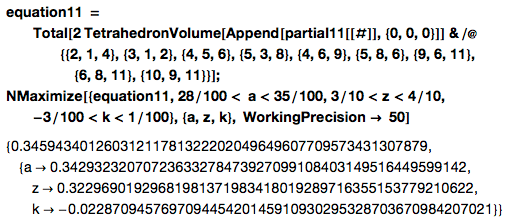Here are two views of 11-BLP.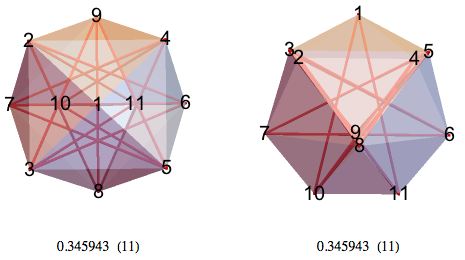Have I really solved these? Maybe not. For these particular symmetries, I’m sure I’ve found the local maximum. For example, here’s a function with a local maximum of 5 at the value 1.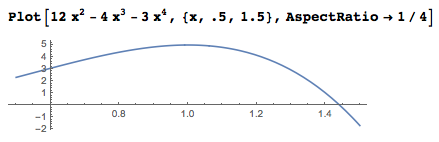Plot a bit more, and the global maximum of 32 can be found at value -2.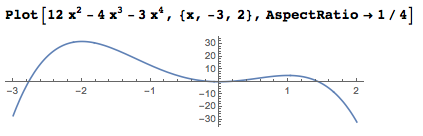In the related Thomson problem, there’s a proof that the 12 vertices of an icosahedron give a minimum energy configuration for 12 electrons. But 7, 8, 9, 10, 11, and 13+ electrons are all considered unsolved. The Kepler conjecture suggested that hexagonal close packing was the densest arrangement of spheres, but a complete formal proof by Thomas Hales wasn’t completed until August 10, 2014. The densest packing of regular tetrahedra, the fraction 4000/4671 = .856347…, wasn’t found until July 27, 2010, and still isn’t proven maximal. Take any solution claims with a grain of salt; geometric maximization problems are notoriously tricky.

For months, my best solution for 11 points was in an asymmetric local maximum. Some (or most) of the following solutions are likely local instead of global, but which ones? With that caveat, we can look at best known solutions for 12 points and above.

12-BLP seems to be the point 12, the slightly messy heptagon 11-6-7-10-8-5-9, and the quadrilateral 1-4-3-2.

13-BLP seems to be the point 13, the slightly messy heptagon 12-8-10-6-7-9-11, and the messy pentagon 1-2-3-4-5.

My attempts to add symmetry have resulted in figures with a lower volume.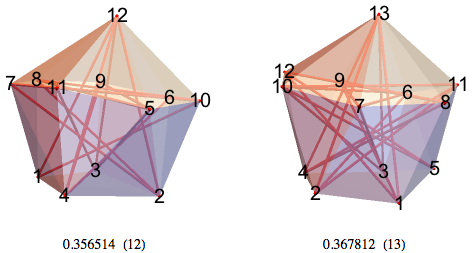So far, my best solutions for a 14-BLP seems to have a lot of symmetry, but I haven’t solved it. I spent some time optimizing a point-heptagon-heptagon solution for a 15-BLP, only to watch my randomizer “improve” it relentlessly by increasing volume while sacrificing symmetry.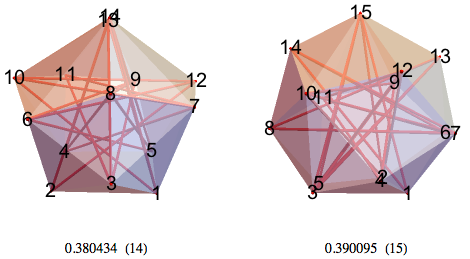17-BLP, 18-BLP—I believe 17-BLP has nice symmetry.

19-BLP, 20-BLP—20 is not the dodecahedron, due to inefficient unit lines through the center.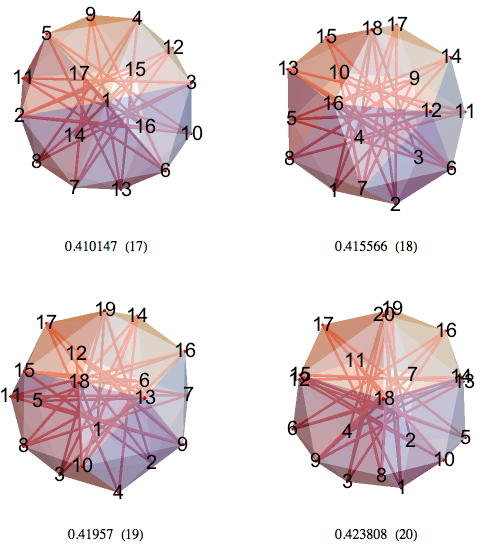The snub cube and half the vertices of the great rhombicuboctahedron both have lower volume than 24-BLP.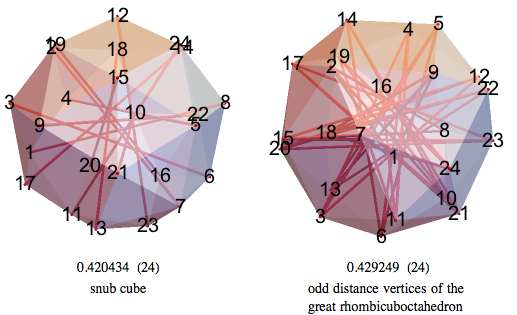21-BLP, 22-BLP—Lots of 7- and 9-pointed stars.

23-BLP, 24-BLP—My best 24-BLP has tetrahedral symmetry.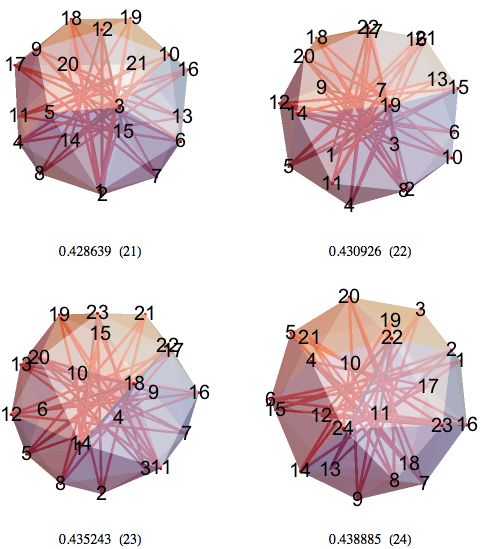Here’s some of the symmetry in the current best 24-BLP. Points 1-12 and 13-24 have respective norms of 0.512593 and 0.515168.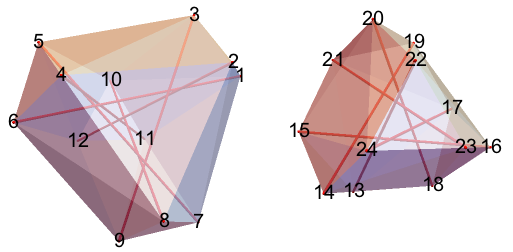16-BLP, 17-BLP—Letting the unit lines define polygons. 16-BLP contains many 7-pointed stars.The same polyhedra shown as solid objects, using ConvexHullMesh[]. That’s BLP 9-10-11-12, 13-14-15-16, 17-18-19-20, 21-22-23-24.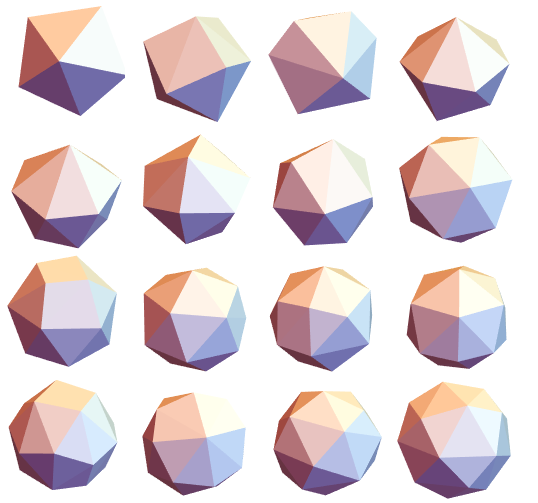Here’s the current table of the best known values.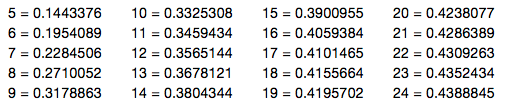Here are the best solutions I’ve found so far for 4 to 24 points.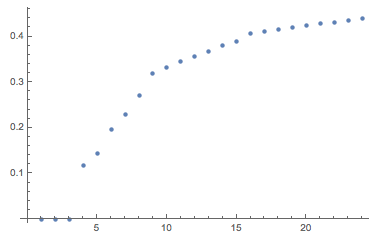Let the points be centered so that the maximal distance from the origin is as small as possible. The below scatterplot shows the distance from the origin for vertices of each polyhedron, from 8 to 24 vertices.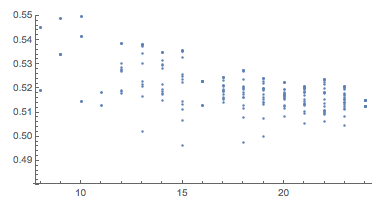Mathematica 10.1 managed to exactly solve 6-BLP, 7-BLP, 8-BLP, 9-BLP, and 16-BLP. It found numerically exact solutions for 10-BLP and 11-BLP, and made good progress for up to 24 points. That gives solutions for seven previously unsolved problems in combinatorial geometry, all by repeating Volume[ConvexHullMesh[points]]. What new features have you had success with?

### References

 B. Kind and P. Kleinschmidt, “On the Maximal Volume of Convex Bodies with Few Vertices,” Journal of Combinatorial Theory, Series A, 21(1) 1976 pp. 124-128.
doi:10.1016/0097-3165(76)90056-X

 A. Klein and M. Wessler, “The Largest Small n-dimensional Polytope with n+3 Vertices,” Journal of Combinatorial Theory, Series A, 102(2), 2003 pp. 401-409.
doi:10.1016/S0097-3165(03)00054-2

 A. Klein and M. Wessler, “A Correction to ‘The Largest Small n-dimensional Polytope with n+3 Vertices,'” Journal of Combinatorial Theory, Series A, 112(1), 2005 pp. 173-174.
doi:10.1016/j.jcta.2005.06.001